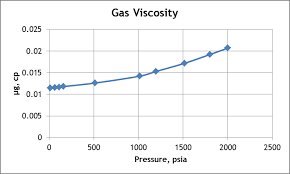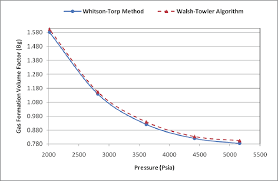## How to Calculate and Solve for MBAL Gas Solubility | The Calculator EncyclopediaThe image above represents the MBAL gas solubility.

To compute for the MBAL gas solubility, four essential parameters are needed and these parameters are Specific Gravity of Solution Gas (γg), Specific Gravity of Stock Tank Oil (γo), Oil FVF (Bo) and Oil Density (ρo).

The formula for calculating MBAL gas solubility:

Rs = Boρo – 62.4γo / 0.0136γg

Where:

Rs = MBAL Gas Solubility
γg = Specific Gravity of Solution Gas
γo = Specific Gravity of Stock Tank Oil
Bo = Oil FVF
ρo = Oil Density

Let’s solve an example;
Given that specific gravity of solution gas is 9, specific gravity of stock tank oil is 13, oil FVF is 19 and oil density is 27. Find the MBAL gas solubility?

This implies that;

γg = Specific Gravity of Solution Gas = 9
γo = Specific Gravity of Stock Tank Oil = 13
Bo = Oil FVF = 19
ρo = Oil Density = 27

Rs = Boρo – 62.4γo / 0.0136γg
Rs = 19 x 27 – 62.4 x 13 / 0.0136 x 9
Rs = 513 – 811.19 / 0.1224
Rs = -298.19 / 0.1224
Rs = -2436.27

Therefore, the MBAL gas solubility is -2436.27.

## How to Calculate and Solve for Gas Formation Volume Factor (FVF) | The Calculator EncyclopediaThe image above represents the gas FVF.

To compute for the gas FVF, three essential parameters are needed and these parameters are Z-Factor (Z), Temperature (T) and Pressure (P).

The formula for calculating the gas FVF:

Bg = 0.00504[ZT / P]

Where;

Bg = Gas FVF
Z = Z-Factor
T = (°R) Temperature
P = Pressure

Let’s solve an example;
Find the Gas FVF when the Z-Factor is 12, temperature is 30 and the pressure is 120.

This implies that;

Z = Z-Factor = 12
T = (°R) Temperature = 30
P = Pressure = 120

Bg = 0.00504[ZT / P]
Bg = 0.00504[12 x 30 / 120]
Bg = 0.00504[360 / 120]
Bg = 0.00504
Bg = 0.01512

Therefore, the gas FVF is 0.01512 bbl / scf.

Calculating for the Z-Factor when the Gas FVF, Temperature and Pressure is Given.

Z = Bg.P / 0.00504T

Where;

Z = Z-Factor
Bg = Gas FVF
T = (°R) Temperature
P = Pressure

Let’s solve an example;
Find the Z-Factor when the Gas FVF is 22, temperature is 16 and the pressure is 80.

This implies that;

Bg = Gas FVF = 22
T = (°R) Temperature = 16
P = Pressure = 80

Z = Bg.P / 0.00504T
Z = 22 x 80 / 0.00504 x 16
Z = 1760 / 0.08064
Z = 21825.39

Therefore, the Z-Factor is 21825.39.

Calculating for the Temperature when the Gas FVF, Z-Factor and the Pressure is Given.

T = Bg.P / 0.00504Z

Where;

T = (°R) Temperature
Z = Z-Factor
Bg = Gas FVF
P = Pressure

Let’s solve an example;
Find the Temperature when the Gas FVF is 44, Z-Factor is 29 and the pressure is 70.

This implies that;

Z = Z-Factor = 29
Bg = Gas FVF = 44
P = Pressure = 70

T = Bg.P / 0.00504Z
T = 44 x 70 / 0.00504 x 29
T = 3080 / 0.14616
T = 21072.79

Therefore, the temperature is 21072.79.